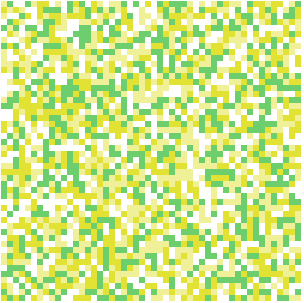Number of pixels: We are modelling the distribution of n2 variables on an n ⨯ n regular square grid. Here you can change n. 50 x 50 Endogenous structure: This is the behaviour of the model when there is no association (i.e., when λ = 0). You can choose one of three scenarios. Pick one and then adjust β0, β1, and β2 to control the model's probabilities under independence (as visualized in the endogenous probability map). Then you can adjust λ to see its effect. The three scenarios are described further in the main text.smoothboxrandom Regression parameters: The parameters in the linear predictor, used to determine the endogenous probabilities. Adjust values by clicking the "−" and "+" buttons. β0 = 0.00    β1 = 0.00    β2 = 0.00 Association parameter: Determines the strength of the influence pixels have on their neighbours. Adjust its value by clicking the "−" and "+" buttons. Positive λ values promote local uniformity. λ = 0.0 Response coding: Set the numeric values used to represent the "low" and "high" states of the binary random variables: either {0, 1} or {−½, ½}. One goal of this simulation is to demonstrate that only the standard model with plus/minus coding gives sensible behaviour. zero/one   plus/minus Model type: Choose the model type, either the standard one or the centered one recommended by Caragea and Kaiser (2009). One goal of this simulation is to demonstrate that only the standard model with plus/minus coding gives sensible behaviour. centered   standard Neighbourhood size: Set the number of neighbours of each pixel: either its four nearest, or eight nearest pixels. 4   8 Update interval: Enter a number of milliseconds to wait between calculating and plotting successive Gibbs sampler iterations. The buttons at right can be used to pause the simulation, run iterations one at a time, resume the simulation, or restart from a random configuration. ms
Probability of the "high" state

0 0.5 1